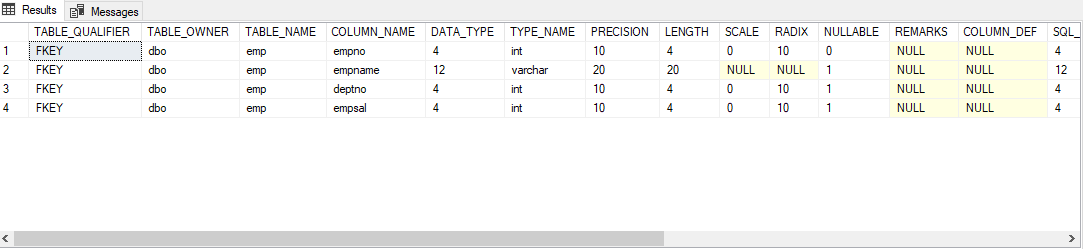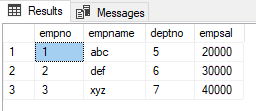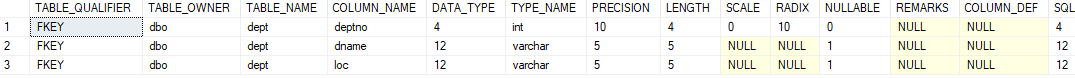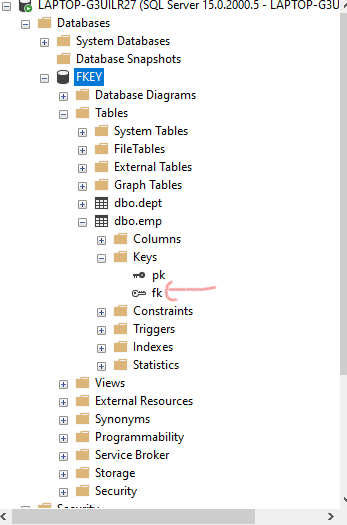Related Articles

# SQL Query to Drop Foreign Key Constraint Using ALTER Command

• Difficulty Level : Medium
• Last Updated : 13 Apr, 2021

Here, we are going to see How to Drop a Foreign Key Constraint using ALTER Command(SQL Query) using  Microsoft SQL Server.

A Foreign key is an attribute in one table which takes references from another table where it acts as the primary key in that table. Also, the column acting as a foreign key should be present in both tables.

### Creating a new Database:

`CREATE DATABASE geeks;`

### Using the Database:

`USE geeks;`

### Table Definition:

We have the following emp table in our database :

```CREATE TABLE emp(
empno number(2) constraint pk primary key ,
empname varchar2(20),
deptno number(2),
empsal number(20));```

To verify table schema use the following query:

`EXEC SP_COLUMNS emp;`

Output:EMP TABLE SCHEMA

Use the below statement to add data to the emp table:

```INSERT INTO emp values(1,'abc',5,20000);
INSERT INTO emp values(2,'def',6,30000);
INSERT INTO emp values(3,'xyz',7,40000);```

Output:VALUES IN EMP TABLE

Now let’s write SQL Query to Drop Foreign Key Constraint Using ALTER Command. For that, we have to create another table called “DEPT”.

### Creating DEPT table

```CREATE TABLE dept(
deptno number(2) constraint pk2 primary key ,
dname varchar2(5),
loc varchar2(5));```

To check out the current table use the following statement:

`SELECT * FROM dept;`

Output:DEPT TABLE SCHEMA

### Adding Data to Dept Table:

Use the below statement to add data to the dept table:

```INSERT INTO dept values(5,'IT','hyd');
INSERT INTO dept values(6,'sales','bglr');
INSERT INTO dept values(7,'mgr','mumb');```

To check out the current table use the following statement:

`SELECT * FROM dept;`

### OutputDEPT TABLE VALUES

Here we have kept the DEPTNO column as common in both EMP and DEPT tables

```ALTER TABLE emp add constraint fk foreign key(deptno) references dept(deptno);
//ADDS FOREIGN KEY CONSTRAINT ON EMP TABLE```FOREIGN KEY “FK” HAS BEEN CREATED

### Dropping  Foreign Constraint:

`ALTER TABLE TABLE NAME drop constraint CONSTRAINT_NAME`

### Removing Foreign key Constraint from the table using ALTER:

`ALTER TABLE emp drop constraint fk;`

Output:Hence, in this way, we can Drop Foreign Key Constraint Using ALTER Command

Attention reader! Don’t stop learning now. Learn SQL for interviews using SQL Course  by GeeksforGeeks.

My Personal Notes arrow_drop_up
Recommended Articles
Page :